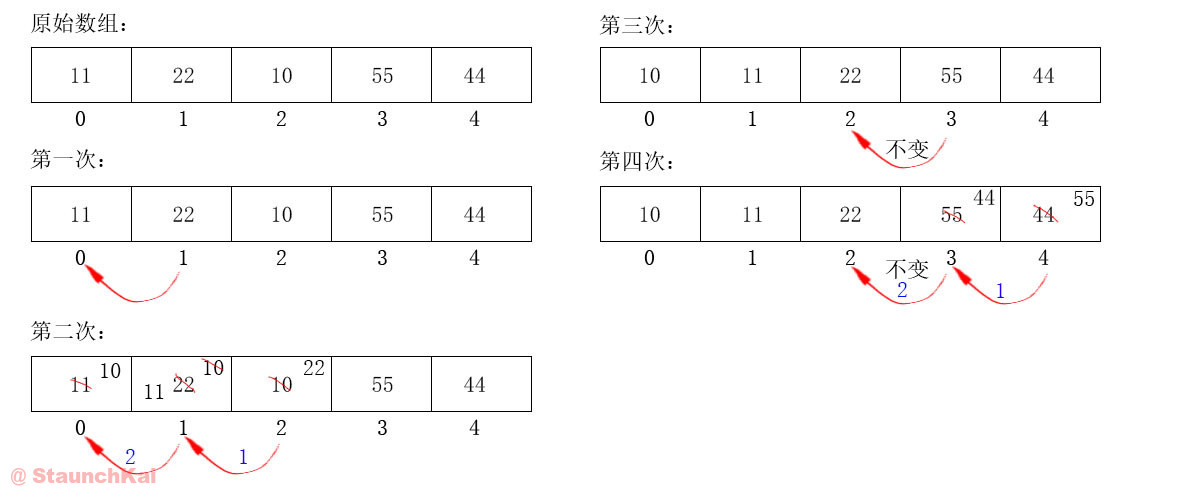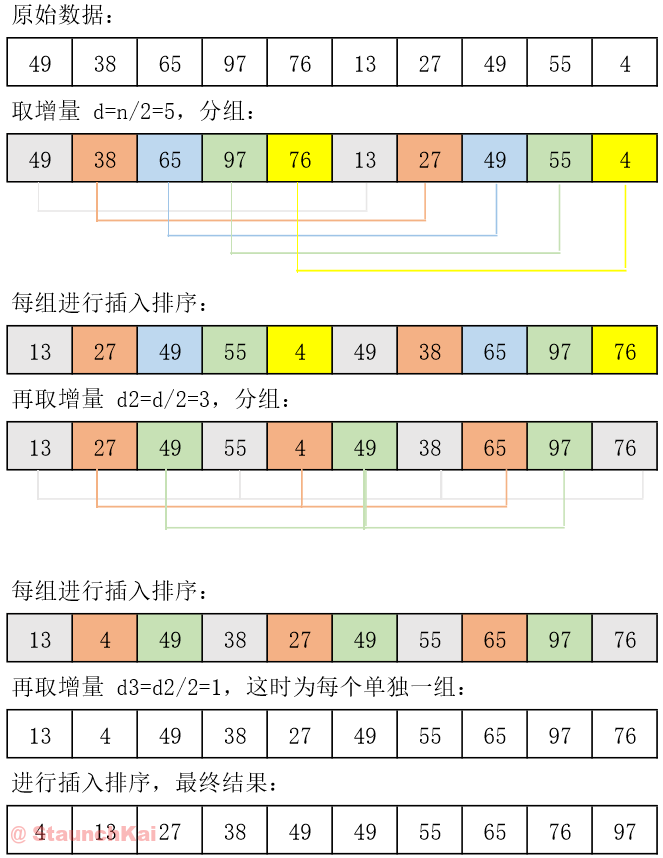# Java 排序之插入排序、希尔排序

## 一、插入排序

### 1. 插入排序算法思想

1) 普遍思想

2) 我的理解与分析

``int[] arr = { 22, 11, 10, 55, 44 };``
• 第 0 个元素 `22` 看作已经排好序；

• 取出第 1 个元素 `11`，在已经排序的元素序列中（第一步的 `22`）从后向前扫描；

• 如果新元素 `11` 比已排序的元素 `22` 小，就把 `22` 往后挪，并将 `11` 插入到前面。这个时候数组应该如下：

``int[] arr = { 11, 22, 10, 55, 44 }``
• 接着在取出第 2 个元素 `10`，与第一个元素 `22` 相比，如果比第一个元素小，就插入到 `22` 之前。这个时候数组应该如下：

``int[] arr = { 11, 10, 22, 55, 44 }``
• `10` 插入后继续与前面的元素相比，小则插入，大则不动。这个时候数组应该如下：

``int[] arr = { 10, 11, 22, 55, 44 }``
• 取出第 3 个元素继续比较……

### 2. 算法图解附视频### 3. 算法代码

``````import java.util.Arrays;

public class InsertSort {
public static void main(String[] args) {
int[] arr = { 11, 22, 10, 55, 44 };
sort(arr);
System.out.println(Arrays.toString(arr));
}

public static void sort(int[] arr) {
for (int i = 1; i < arr.length; i++) {  // 从第一个元素开始遍历
for (int j = i; (j > 0) && (arr[j] < arr[j - 1]); j--) {    // 条件：是否比前一个元素小，j-- 是为了挪位后再次与前一个元素比较
int temp = arr[j];      // 把移动的元素暂时存起来
arr[j] = arr[j - 1];    // 把大的元素往后挪
arr[j - 1] = temp;      // 把小的元素放到前面
}
}
}
}``````

## 二、希尔排序

### 2. 算法分析

``int[] arr = { 49, 38, 65, 97, 76, 13, 27, 49, 55, 04 };``
• 先取一个增量 d = n/2 = 5，把每相隔 5 的数据分为一组，如下：

``````第一组 ---> 49 -- 13
第二组 ---> 38 -- 27
第三组 ---> 65 -- 49
第四组 ---> 97 -- 55
第五组 ---> 76 -- 04``````
• 每一组进行插入排序，如下：

``````第一组 ---> 13 -- 49
第二组 ---> 27 -- 38
第三组 ---> 49 -- 65
第四组 ---> 55 -- 97
第五组 ---> 04 -- 76``````
• 第一次的结果为：

``{ 13, 27, 49, 55, 04, 49, 38, 65, 97, 76 };``
• 取第二个增量 d2 = d/2 = 3，把每相隔 3 的数据分为一组，如下：

``````第一组 ---> 13 -- 55 -- 38 -- 76
第二组 ---> 27 -- 04 -- 65
第三组 ---> 49 -- 49 -- 97``````
• 每一组进行插入排序，如下：

``````第一组 ---> 13 -- 38 -- 55 -- 76
第二组 ---> 04 -- 27 -- 65
第三组 ---> 49 -- 49 -- 97``````
• 第二次的结果为：

``{ 13, 04, 49, 38, 27, 49, 55, 65, 97, 76 };``
• 取第三个增量 d3 = d2/2 = 1，把每相隔 1 的数据分为一组，这时开始直接插入排序即可，结果如下：

``{ 04, 13, 27, 38, 49, 49, 55, 65, 76, 97 };``

### 3. 算法图解附视频### 4. 算法代码

``````import java.util.Arrays;

public class ShellSort {
public static void main(String[] args) {
int[] arr = { 49, 38, 65, 97, 76, 13, 27, 49, 55, 04 };
sort(arr);
System.out.println(Arrays.toString(arr));
}

public static void sort(int[] arr) {
for (int d = arr.length / 2; d > 2; d /= 2) {
for (int i = 0; i < d; i++) {
insertSort(arr, i, d);
}
}
insertSort(arr, 0, 1);
}

// 插入排序
public static void insertSort(int[] arr, int i, int d) {
for (int a = i + d; a < arr.length; a += d) {    // a += d
for (int j = a; (j >= d) && (arr[j] < arr[j - d]); j -= d) {
int temp = arr[j];
arr[j] = arr[j - d];
arr[j - d] = temp;
}
}
}
}
``````

×